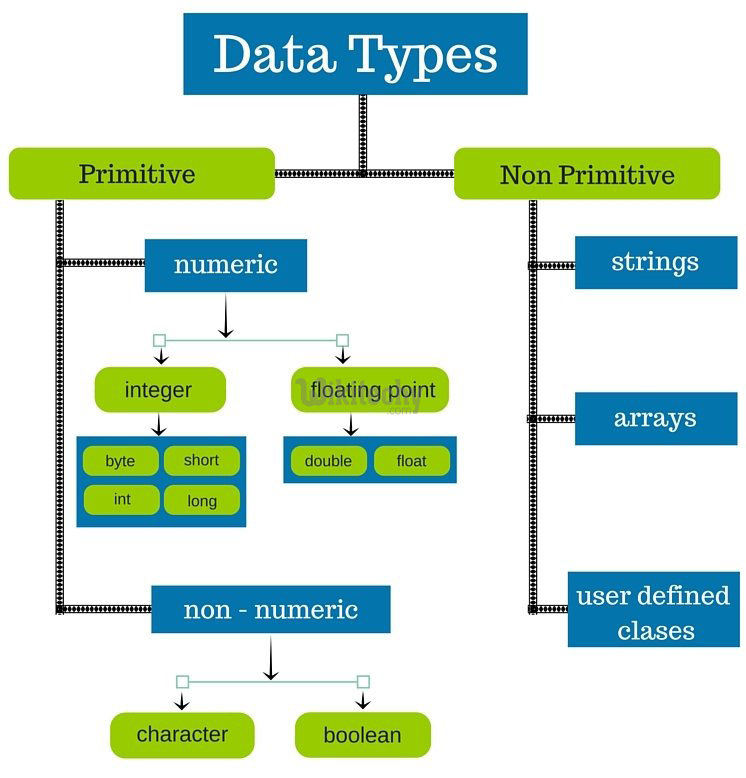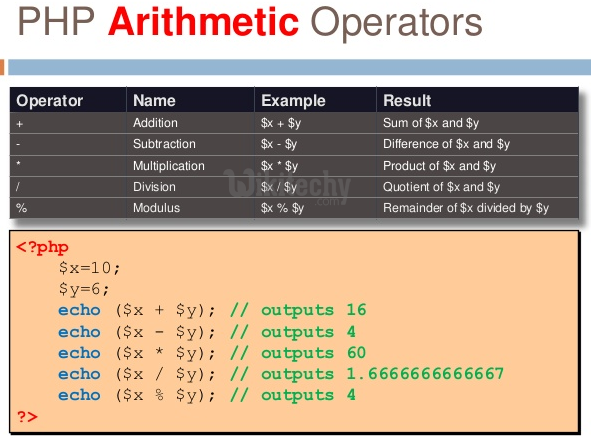# php tutorial - PHP Data Types - php programming - learn php - php code - php script

• The main approach of data types is to store the data in the PHP program by using a variable.
• PHP supports the following data types:## Scalar types :

• boolean
• integer
• float
• string• array
• object

• resources
• NULL

## Boolean values :

• Boolean is the simplest data type. It’s like a switch that has only two states ON means true(1) and OFF means false(0).

## Integers :

• Integer means numeric data types. A whole number with no fractional & decimal component will be there.
• Integer may be lesser than or greater than or sometimes equals to zero.

## Floating point numbers :

• A floating point number is a number with a decimal point or a number in exponential form.

## Strings :

• A string is series of characters, where a character is the same as a byte.
• This means that PHP only supports a 256-character set, and hence does not offer native Unicode support.

## Array :

• An array stores several values in one single variable.

## Object :

• An object is a data type which stores data and information on how to process that data.

## Resources :

• The resources are special variables that holds the references to resources which is external to PHP such as database connections.

## NULL :

• The NULL is defined as a special variable. Mostly, the data type which means non existent or not known or else empty.